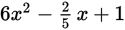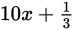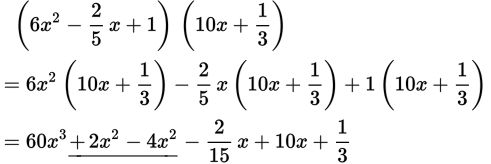# SAT Math Multiple Choice Question 309: Answer and Explanation

### Test Information

Question: 309

9. What is the coefficient of x2 whenis multiplied by?

• A. -4
• B. -2
• C. 2
• D. 4

Explanation:

B

Difficulty: Medium

Category: Passport to Advanced Math / Exponents

Strategic Advice: When multiplying polynomials, carefully multiply each term in the first factor by each term in the second factor. This question doesn't ask for the entire product, so check to make sure you answered the right question (the coefficient of x2).